## 数据结构与算法二 算法分析

article/2023/9/24 22:26:58

## 1.1 算法的时间复杂度分析

``````public static void main(String[] args) {long start = System.currentTimeMillis();int sum = 0;int n=100;for (int i = 1; i <= n; i++) {sum += i;}System.out.println("sum=" + sum);long end = System.currentTimeMillis();System.out.println(end-start);
}
``````

1. 算法采用的策略和方案；
2. 编译产生的代码质量；
3. 问题的输入规模(所谓的问题输入规模就是输入量的多少)；
4. 机器执行指令的速度；

``````//如果输入量为n为1，则需要计算1次；
//如果输入量n为1亿，则需要计算1亿次；
public static void main(String[] args) {int sum = 0;//执行1次int n=100;//执行1次for (int i = 1; i <= n; i++) {//执行了n+1次sum += i;//执行了n次}System.out.println("sum=" + sum);
}
``````

``````//如果输入量为n为1，则需要计算1次；
//如果输入量n为1亿，则需要计算1次；
public static void main(String[] args) {int sum = 0;//执行1次int n=100;//执行1次sum = (n+1)*n/2;//执行1次System.out.println("sum="+sum);
}
``````

``````public static void main(String[] args) {int sum=0;int n=100;for (int i = 1; i <=n ; i++) {for (int j = 1; j <=n ; j++) {sum+=i;}}System.out.println("sum="+sum);
}
``````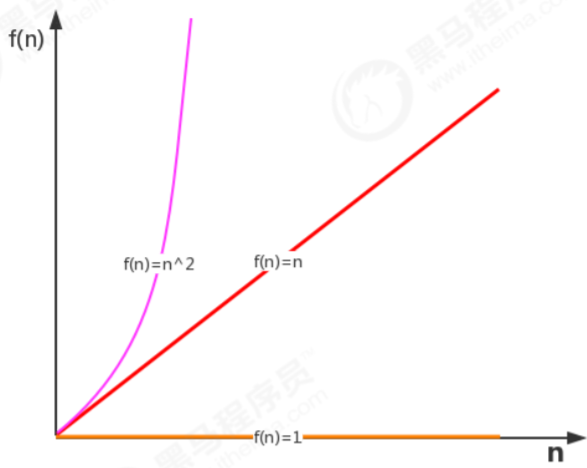### 1.1.1 函数渐近增长

1. 算法A1要做2n+3次操作，可以这么理解：先执行n次循环，执行完毕后，再有一个n次循环，最后有3次运算；
2. 算法A2要做2n次操作；
3. 算法B1要做3n+1次操作，可以这样理解：先执行n次循环，再执行一个n次循环，再执行一个n次循环，最后有1次运算。
4. 算法B2要做3n次操作；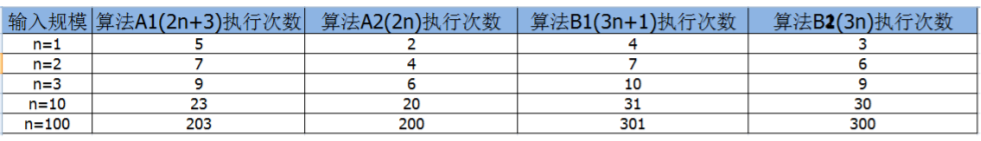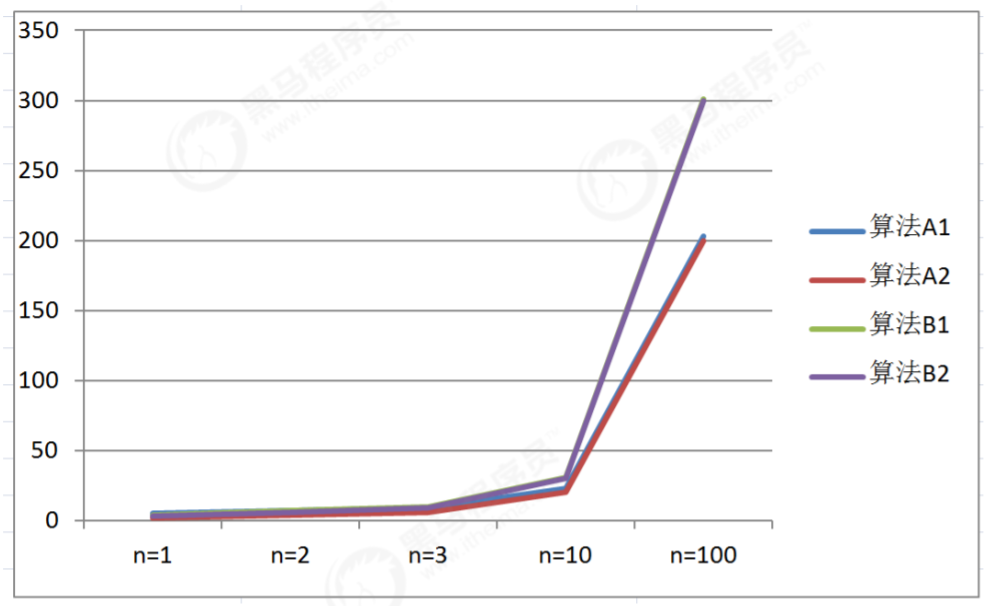1. 算法C1需要做4n+8次操作
2. 算法C2需要做n次操作
3. 算法D1需要做2n^2次操作
4. 算法D2需要做n^2次操作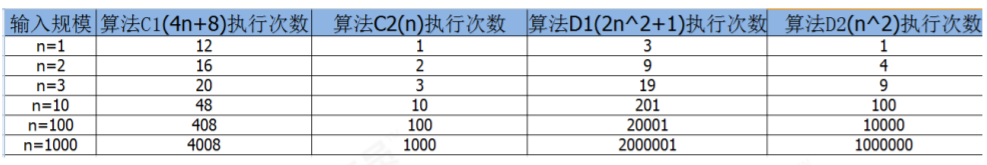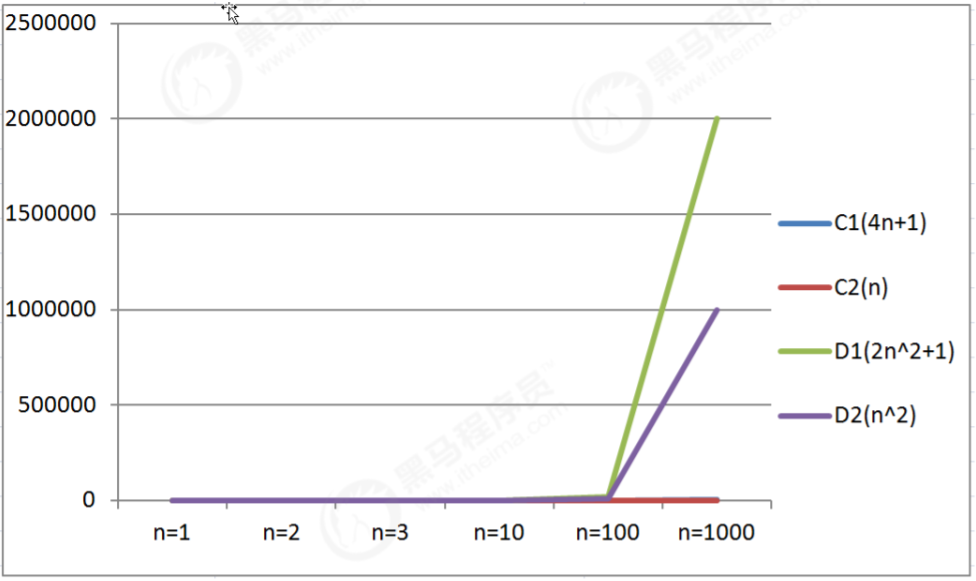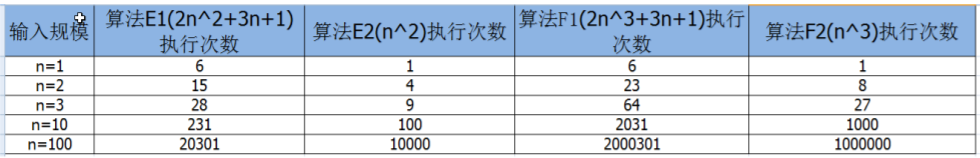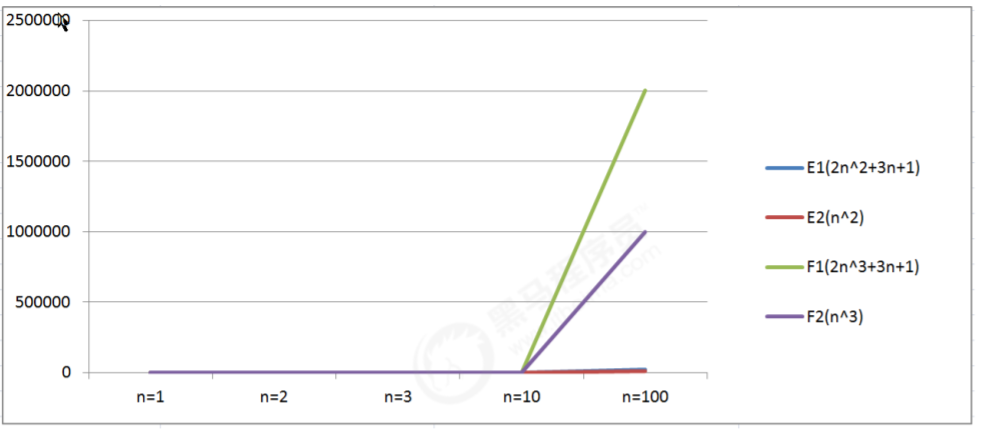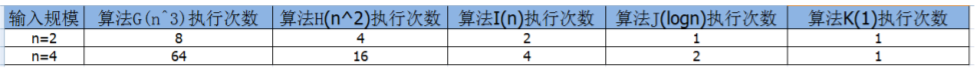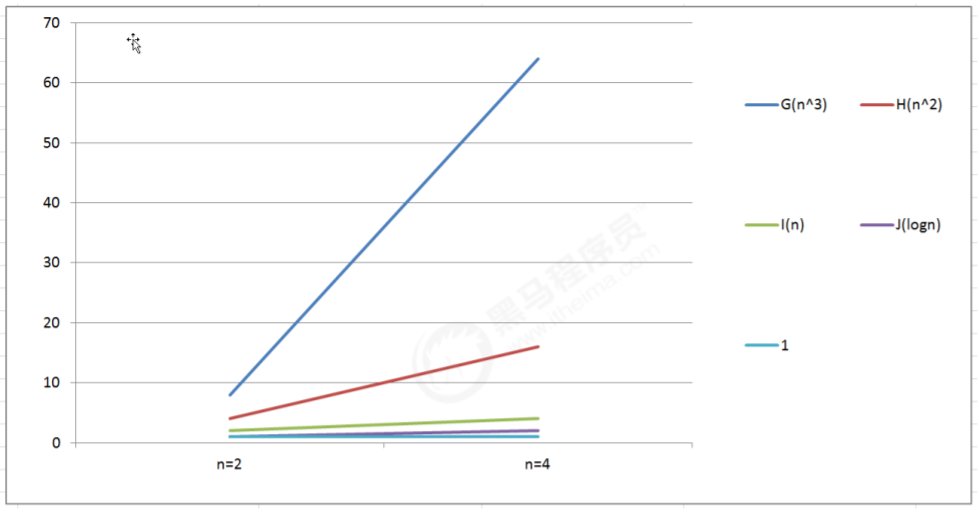1. 算法函数中的常数可以忽略；
2. 算法函数中最高次幂的常数因子可以忽略；
3. 算法函数中最高次幂越小，算法效率越高。

### 1.1.2 算法时间复杂度

#### 1.1.2.1 大O记法

``````public static void main(String[] args) {int sum = 0;//执行1次int n=100;//执行1次sum = (n+1)*n/2;//执行1次System.out.println("sum="+sum);
}
``````

``````public static void main(String[] args) {int sum = 0;//执行1次int n=100;//执行1次for (int i = 1; i <= n; i++) {sum += i;//执行了n次}System.out.println("sum=" + sum);
}
``````

``````public static void main(String[] args) {int sum=0;//执行1次int n=100;//执行1次for (int i = 1; i <=n ; i++) {for (int j = 1; j <=n ; j++) {sum+=i;//执行n^2次}}System.out.println("sum="+sum);
}
``````

1. 用常数1取代运行时间中的所有加法常数；
2. 在修改后的运行次数中，只保留高阶项；
3. 如果最高阶项存在，且常数因子不为1，则去除与这个项相乘的常数；

#### 1.1.2.2 常见的大O阶

1.线性阶

``````public static void main(String[] args) {int sum = 0;int n=100;for (int i = 1; i <= n; i++) {sum += i;}System.out.println("sum=" + sum);
}
``````

2.平方阶

``````public static void main(String[] args) {int sum=0,n=100;for (int i = 1; i <=n ; i++) {for (int j = 1; j <=n ; j++) {sum+=i;}}System.out.println(sum);
}
``````

3.立方阶

``````public static void main(String[] args) {int x=0,n=100;for (int i = 1; i <=n ; i++) {for (int j = i; j <=n ; j++) {for (int j = i; j <=n ; j++) {x++;}}}System.out.println(x);
}
``````

4.对数阶

``````int i=1,n=100;
while(i<n){i = i*2;
}
``````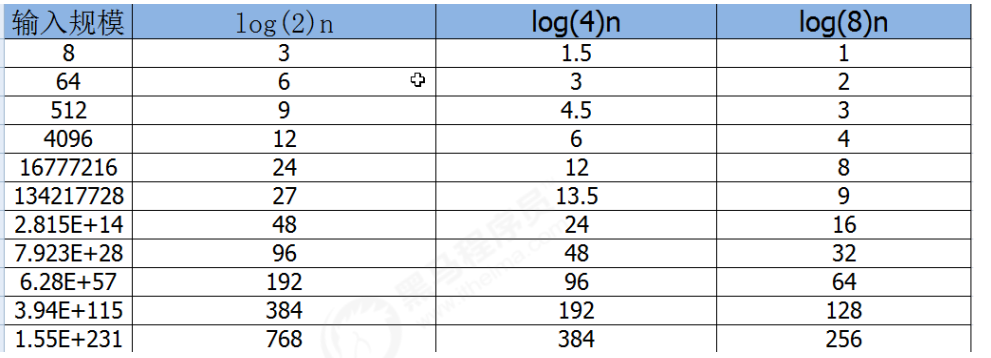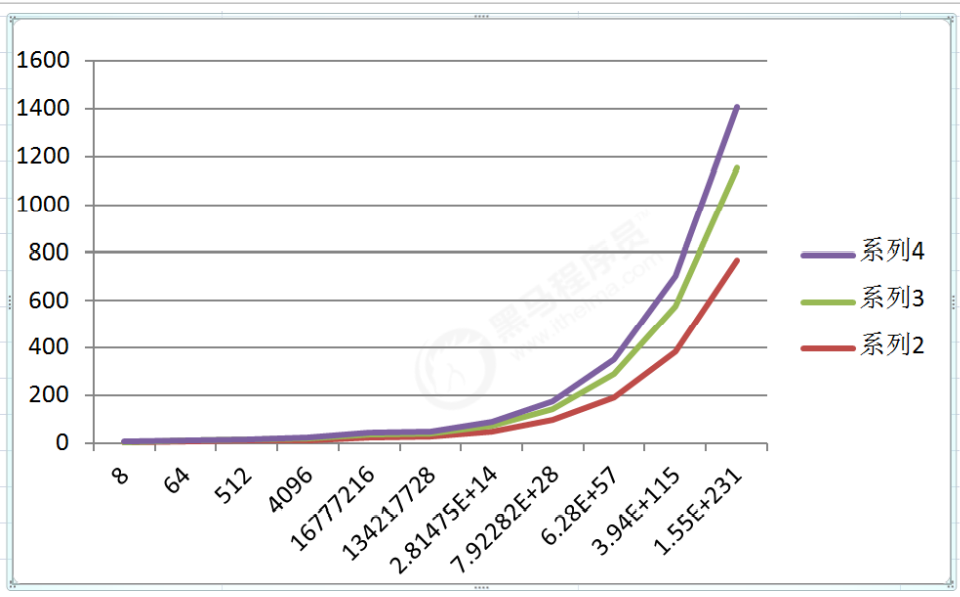5.常数阶

``````public static void main(String[] args) {int n=100;int i=n+2;System.out.println(i);
}
``````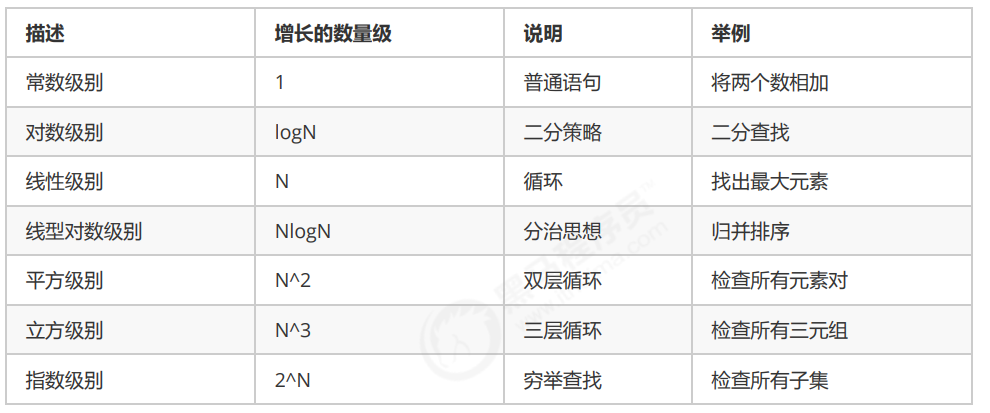`````` O(1)<O(logn)<O(n)<O(nlogn)<O(n^2)<O(n^3)
``````

#### 1.1.2.3 函数调用的时间复杂度分析

``````public static void main(String[] args) {int n=100;for (int i = 0; i < n; i++) {show(i);}
}private static void show(int i) {System.out.println(i);
}
``````

``````public static void main(String[] args) {int n=100;for (int i = 0; i < n; i++) {show(i);}
}private static void show(int i) {for (int j = 0; j < i; i++) {System.out.println(i);}
}
``````

``````public static void main(String[] args) {int n=100;show(n);for (int i = 0; i < n; i++) {show(i);}for (int i = 0; i < n; i++) {for (int j = 0; j < n; j++) {System.out.println(j);}}
}private static void show(int i) {for (int j = 0; j < i; i++) {System.out.println(i);}
}
``````

#### 1.1.2.4 最坏情况

``````public int search(int num){int[] arr={11,10,8,9,7,22,23,0};for (int i = 0; i < arr.length; i++) {if (num==arr[i]){return i;}}return -1;
}
``````

## 1.2 算法的空间复杂度分析

### 1.2.1 java中常见内存占用

1.基本数据类型内存占用情况：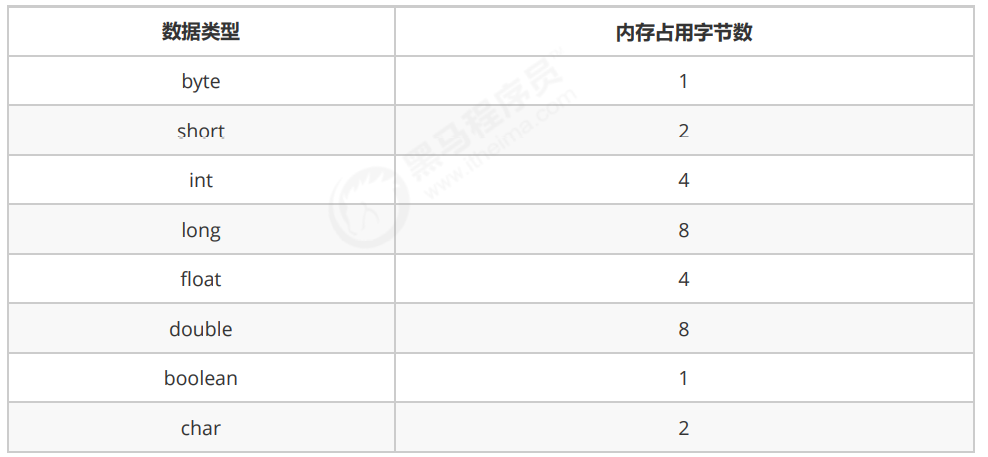2.计算机访问内存的方式都是一次一个字节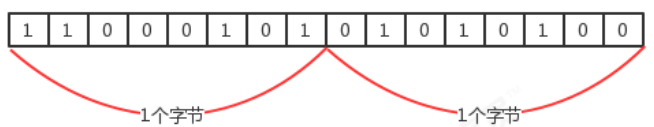3.一个引用（机器地址）需要8个字节表示：

4.创建一个对象，比如new Date()，除了Date对象内部存储的数据(例如年月日等信息)占用的内存，该对象本身也有内存开销，每个对象的自身开销是16个字节，用来保存对象的头信息。

5.一般内存的使用，如果不够8个字节，都会被自动填充为8字节：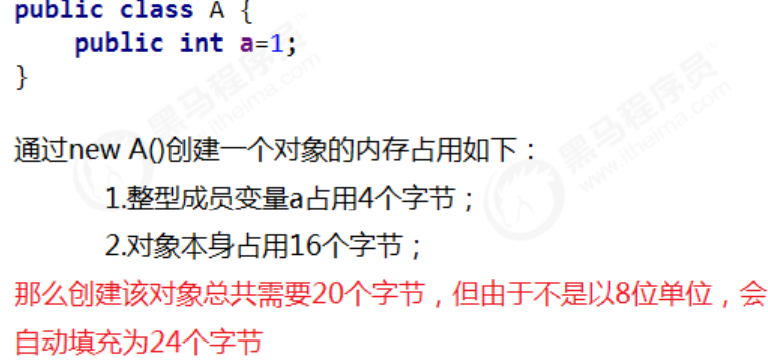6.java中数组被被限定为对象，他们一般都会因为记录长度而需要额外的内存，一个原始数据类型的数组一般需要24字节的头信息(16个自己的对象开销，4字节用于保存长度以及4个填充字节)再加上保存值所需的内存。

### 1.2.2 算法的空间复杂度

``````public static int[] reverse1(int[] arr){int n=arr.length;//申请4个字节int temp;//申请4个字节for(int start=0,end=n-1;start<=end;start++,end--){temp=arr[start];arr[start]=arr[end];arr[end]=temp;}return arr;
}
``````

``````public static int[] reverse2(int[] arr){int n=arr.length;//申请4个字节int[] temp=new int[n];//申请n*4个字节+数组自身头信息开销24个字节for (int i = n-1; i >=0; i--) {temp[n-1-i]=arr[i];}return temp;
}
``````

4+4n+24=4n+28;

### OceanBase CTO杨传辉：持续降低使用门槛，打造开发者友好的分布式数据库

3月25日&#xff0c;首届OceanBase开发者大会在北京举行。大会发布了OceanBase 4.1版本&#xff0c;公布两大友好工具&#xff0c;升级文档易用性&#xff0c;统一企业版和社区版代码分支&#xff0c;全面呈现了OceanBase打造极致的开发者友好数据库的成果。过去13年&#xff0…